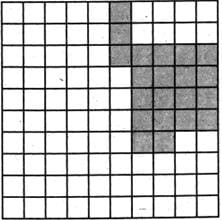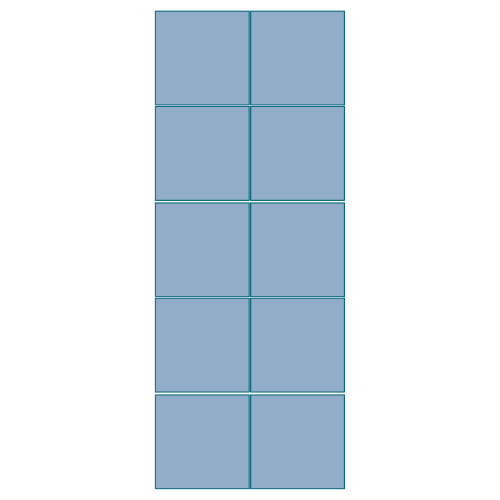Test: Area & Perimeter - 1

# Test: Area & Perimeter - 1

Test Description

## 10 Questions MCQ Test Mathematics for Class 5 | Test: Area & Perimeter - 1

Test: Area & Perimeter - 1 for Class 5 2023 is part of Mathematics for Class 5 preparation. The Test: Area & Perimeter - 1 questions and answers have been prepared according to the Class 5 exam syllabus.The Test: Area & Perimeter - 1 MCQs are made for Class 5 2023 Exam. Find important definitions, questions, notes, meanings, examples, exercises, MCQs and online tests for Test: Area & Perimeter - 1 below.
Solutions of Test: Area & Perimeter - 1 questions in English are available as part of our Mathematics for Class 5 for Class 5 & Test: Area & Perimeter - 1 solutions in Hindi for Mathematics for Class 5 course. Download more important topics, notes, lectures and mock test series for Class 5 Exam by signing up for free. Attempt Test: Area & Perimeter - 1 | 10 questions in 10 minutes | Mock test for Class 5 preparation | Free important questions MCQ to study Mathematics for Class 5 for Class 5 Exam | Download free PDF with solutions
 1 Crore+ students have signed up on EduRev. Have you?
Test: Area & Perimeter - 1 - Question 1

### What is the area of rectangle if Length = 2m, Breadth = 5m

Detailed Solution for Test: Area & Perimeter - 1 - Question 1

The area of rectangle is given by length × breadth. So the area is 2 × 5 = 10m2.

Test: Area & Perimeter - 1 - Question 2

### The third side of the triangle if the perimeter of a triangle is 20m and two of its sides are 5m and 4m, is

Detailed Solution for Test: Area & Perimeter - 1 - Question 2

Perimeter of a triangle =1stside+2ndside+3rd side So, 20=5+4+3rdside 3rdside=20−9=11m.

Test: Area & Perimeter - 1 - Question 3

### Find the area of shaded region. (Each square = 1m2)Detailed Solution for Test: Area & Perimeter - 1 - Question 3

Calculate no. of shaded boxes. It comes out to be 21. And each box has area of 1m2. So the area of shaded region becomes21m2.

Test: Area & Perimeter - 1 - Question 4

The difference between the length and the breadth of a rectangle is 8 cm and the perimeter is 64 cm. Which of the following can be the length and breadth of this rectangle?

Detailed Solution for Test: Area & Perimeter - 1 - Question 4

Length of rectangle = x cm Breadth of rectangle = (x - 8) cm Perimeter of rectangle = 2(x + x - 8) = 2(2x - 8) Given 2(2x - 8) = 64 ⇒  2x = 40  ⇒  x = 20 L = 20, B = 20 - 8 = 12

Test: Area & Perimeter - 1 - Question 5

The area of a square is 100 sq. cm. If the sides this square are increased by 10% then what will be the area of new square?

Detailed Solution for Test: Area & Perimeter - 1 - Question 5

Given area of square = 100 side×side=100=10×10 ⇒Side = 10 cm Increased in side of square = 10% of 10 = 1 ∴ New side = 10 + 1 = 11 ∴ Area of new square =(11)2=121sq.cm.

Test: Area & Perimeter - 1 - Question 6

The area of a square is equal to the area of a rectangle of I = 8 cm and b = 2 cm. What is the side of the square?

Detailed Solution for Test: Area & Perimeter - 1 - Question 6

Area of rectangle = l×b

l = 8cm,. b= 2 cm

Area of rectangle= ( 8×2)cm

= 16cm^2

Area of rectangle= Area of square

therefore area of the square is 16cm^2

Area of square= (a)^2

√16 = a

Side of the square 4cm

Test: Area & Perimeter - 1 - Question 7

A cuboid measures 24m × 12m × 10m. how many cubes of side 3m can fit in the box?

Detailed Solution for Test: Area & Perimeter - 1 - Question 7

Given : length = 24 m , breadth= 12 m , height = 10 m
edge of a cube = 3 m
to find how many cubes of side 3 m can fit in the cuboid, let us first find the volume of the cuboid:

volume of the cuboid = l × b × h ⇒ 24×12×10 = 2880 m³

now, volume of cube :
vol of cube = a³ (where 'a' is the side )  ⇒ 3³ = 27 m³

no. of cubes that can fit in the cuboid = volume of the cuboid ÷ volume of the                                                                                                                  cube
⇒ 2880 ÷ 27 = 106.66 ⇒ 106 cubes

Test: Area & Perimeter - 1 - Question 8

Calculate the area of rectangle given below if each square has an area of 4m2.Detailed Solution for Test: Area & Perimeter - 1 - Question 8

There are 2×5=10 squares and each square has an area of 4m2 So, the area of rectangle =2×5×4=40m2.

Test: Area & Perimeter - 1 - Question 9

Area of rectangle is 32m2. If the length is 8m then breadth = ________

Detailed Solution for Test: Area & Perimeter - 1 - Question 9

Test: Area & Perimeter - 1 - Question 10

If a figure has sides 4m, 2m, 4m and 2m then which type of figure is this?

Detailed Solution for Test: Area & Perimeter - 1 - Question 10

There are four sides .So it cannot be circle or triangle. Now, it has 2 pairs of equal sides. So it is a rectangle as the square has all its four sides equal.

## Mathematics for Class 5

12 videos|55 docs|35 tests
 Use Code STAYHOME200 and get INR 200 additional OFF Use Coupon Code
Information about Test: Area & Perimeter - 1 Page
In this test you can find the Exam questions for Test: Area & Perimeter - 1 solved & explained in the simplest way possible. Besides giving Questions and answers for Test: Area & Perimeter - 1, EduRev gives you an ample number of Online tests for practice

## Mathematics for Class 5

12 videos|55 docs|35 tests